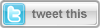(X) Hide this
• Join
• Generate New Image

# Simulating rain in Silverlight Part 3 - Adding wind effectLazar Nikolov
Joined Dec 15, 2010
Articles:   7
More Articles
0 comments   /   posted on Sep 28, 2011
Tags:   lazar-nikolov
Categories:   Gaming , General , Design### Introduction

This is the logical continuation of my article "Simulating rain in Silverlight Part 2 - Optimization".

### More resources...

All SilverlightShow EbooksIn this publication, I will add wind effect to the rain. And why is this wind effect the logical extension of the rain? Obviously, the nature rarely brings us the rain without a wind. Furthermore, it is not that trivial to add the wind effect...

Ок, let's take a good look at the phenomenon, just to make sure we have a solid understanding of what we're trying to simulate. Our simulation will assume the wind is constant. This way we avoid the random fluctuations of the rain drops. Why is this? Since Silverlight does not limit you, just experiment with it by yourself. Clear, we have rain drops, constant wind blowing the scene, and that is...just deal with the angle the drops are falling.

From this point on, I will expect you to have read my previous articles, "Simulating rain in Silverlight" and "Simulating rain in Silverlight Part 2 - Optimization" since I am going to build over them.

Before I begin with the problem, here is a demo:

View live demo - 30 degrees angle

### What is the problem?

What are we up to now? In the previous articles we have the rain with the falling straight down drops. Now we will alter the angle of the drops to simulate the wind effect. You would say “Okay, that’s easy, just change the angle in the RotateTransform in the algorithm and it’s over”. I wish it was that easy.

Changing the angle in the transformation does actually resemble the wind effect, but having the scene in the Canvas, we have to slice two portions to keep the picture consistent. First, we have drops from the left of the scene that need to be hidden after reaching the scene border. And then, we have the drops from the right that need to be hidden until reached the scene border.

And,

### What is the solution?

Not that hard, actually, I promise. Just simple math...trigonometry.

We have three types of drops in this simulation. One that falls all its way in the scene. Other that starts in the scene and falls until hits the left border of the scene. And last, the drops that start their way outside the scene and, at some moment, enter the scene until they fall.

We don’t need to pay any special attention to the first type of drops since they just need to have their angle set. It is rather different with the rest of the drops. First, we need to calculate what the point in the scene that distinguishes the first from the second type is. I call this property Slice. Having the height of the scene and the angle you simply use the tangent of the angle. Based on Slice, I need the slice coefficient:

`this``.Slice = ( ``int` `)( height * Math.Tan( Math.PI * ``this``.Angle / 180 ) );`
`this``.sliceCoef = ( ``this``.FallDistance * Math.Sin( Math.PI * ``this``.Angle / 180 ) ) / ``this``.Width;`

Having this information, it is easy to determine what type is a drop. Of course, the third type is easily distinguishable using the right border of the scene.

`int` `leftMargin = Convert.ToInt32( rand.NextDouble() * ( ``this``.Width + ``this``.Slice ) );`
`this``.sceneDrops[ currentDrop ].Margin = ``new` `Thickness( leftMargin, 0, 0, 0 );`
`double` `coef = ( ``double` `)leftMargin / ( ``double` `)``this``.Width;`

The drop is Type 1 if the coefficient is less than the slice coefficient:

`if` `( coef <= ``this``.sliceCoef )`
`{`
`    ``int` `newFinalHeight = ( ``int` `)( ( leftMargin ) / ( Math.Sin( Math.PI * ``this``.Angle / 180 ) ) );`
`    ``this``.sceneDrops[ currentDrop ].FallDistance = newFinalHeight;`
`    ``this``.sceneDrops[ currentDrop ].FallTime =`
`        ``TimeSpan.FromMilliseconds( Constants.ANIMATION_DURATION.TotalMilliseconds *`
`        ``( ( ``double` `)newFinalHeight / ( ``double` `)``this``.DropSettings.FallDistance ) );`
`}`

To calculate the new length of the fall, that is the fall height, we use trigonometry. Of course, we also must alter the duration of the fall.

Similarly, to calculate the way of Type 3 drops, we use this code:

`if` `( coef >= 1 )`
`{`
`    ``int` `newInitialHeight = ( ``int` `)( ( ``double` `)( leftMargin - ``this``.Width ) / Math.Sin( Math.PI * ``this``.Angle / 180 ) );`
`    ``this``.sceneDrops[ currentDrop ].FallInitialHeight = newInitialHeight;`
`    ``this``.sceneDrops[ currentDrop ].FallTime =`
`        ``TimeSpan.FromMilliseconds( Constants.ANIMATION_DURATION.TotalMilliseconds *`
`        ``( ( ``double` `)( ``this``.FallDistance - newInitialHeight ) / ( ``double` `)``this``.FallDistance ) );`
`}`

And voila! That was the main part of it all. Wasn’t that hard, right?

### Conclusion

To summarize, in this article we’ve simulated wind effect applied to the rain effect from my previous article - "Simulating rain in Silverlight Part 2 - Optimization". In my next article, I will add visuals to harness and change the speed of the wind through UI. I will also create sound effects for both wind and rain to “color” the scene. J

*      *